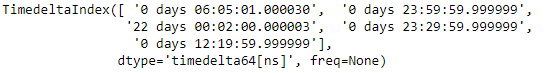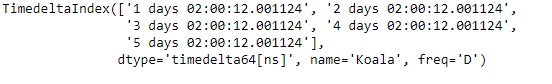GeeksforGeeks App
Open AppBrowser
Continue

# Python | Pandas TimedeltaIndex.transpose()

Python is a great language for doing data analysis, primarily because of the fantastic ecosystem of data-centric python packages. Pandas is one of those packages and makes importing and analyzing data much easier.

Pandas TimedeltaIndex.transpose() function return the transpose of the given TimedeltaIndex object, which is by definition self.

Syntax : TimedeltaIndex.transpose(*args, **kwargs)
Parameters : *args, **kwargs
Return : Object of same type

Example #1: Use TimedeltaIndex.transpose() function to find the transpose of the given TimedeltaIndex object.

## Python3

 `# importing pandas as pd``import` `pandas as pd` `# Create the TimedeltaIndex object``tidx ``=` `pd.TimedeltaIndex(data ``=``[``'06:05:01.000030'``, ``'+23:59:59.999999'``,``                        ``'22 day 2 min 3us 10ns'``, ``'+23:29:59.999999'``,``                        ``'+12:19:59.999999'``])` `# Print the TimedeltaIndex object``print``(tidx)`

Output :Now we will use the TimedeltaIndex.transpose() function to find the transpose of the tidx object.

## Python3

 `# find transpose``tidx.transpose()`

Output :As we can see in the output, the TimedeltaIndex.transpose() function has returned the transpose of the tidx object which is by definition self.

Example #2: Use TimedeltaIndex.transpose() function to find the transpose of the given TimedeltaIndex object.

## Python3

 `# importing pandas as pd``import` `pandas as pd` `# Create the TimedeltaIndex object``tidx ``=` `pd.TimedeltaIndex(start ``=``'1 days 02:00:12.001124'``,``                        ``periods ``=` `5``, freq ``=``'D'``, name ``=``'Koala'``)` `# Print the TimedeltaIndex object``print``(tidx)`

Output :Now we will use the TimedeltaIndex.transpose() function to find the transpose of the tidx object.

## Python3

 `# find transpose``tidx.transpose()`

Output :As we can see in the output, the TimedeltaIndex.transpose() function has returned the transpose of the tidx object which is by definition self.

My Personal Notes arrow_drop_up